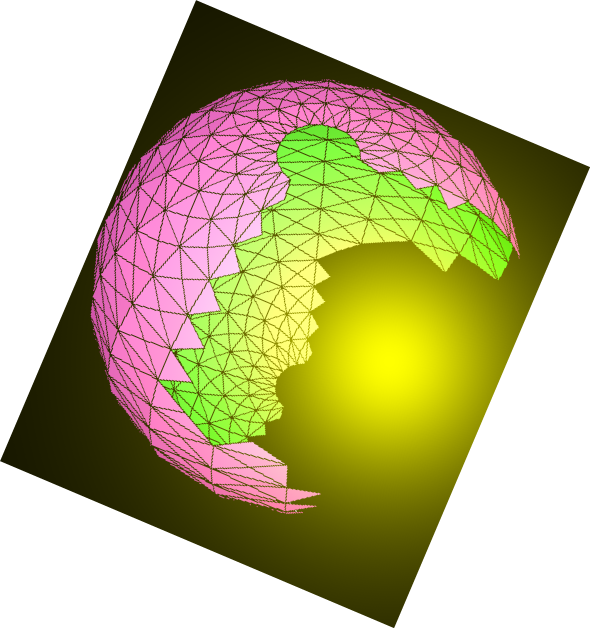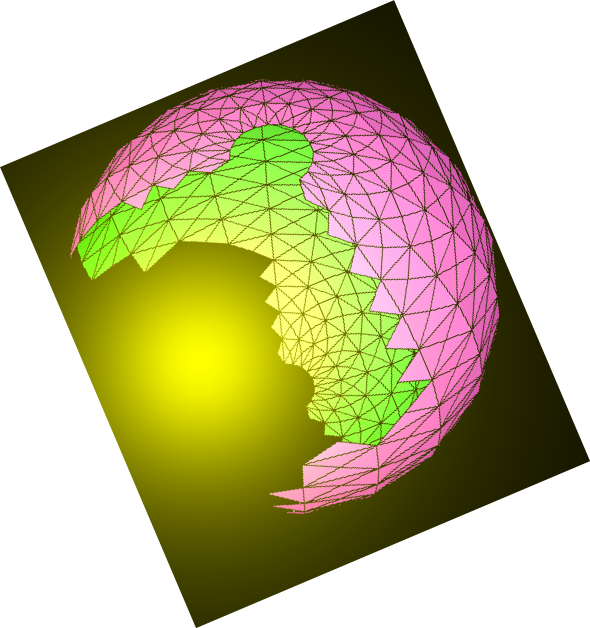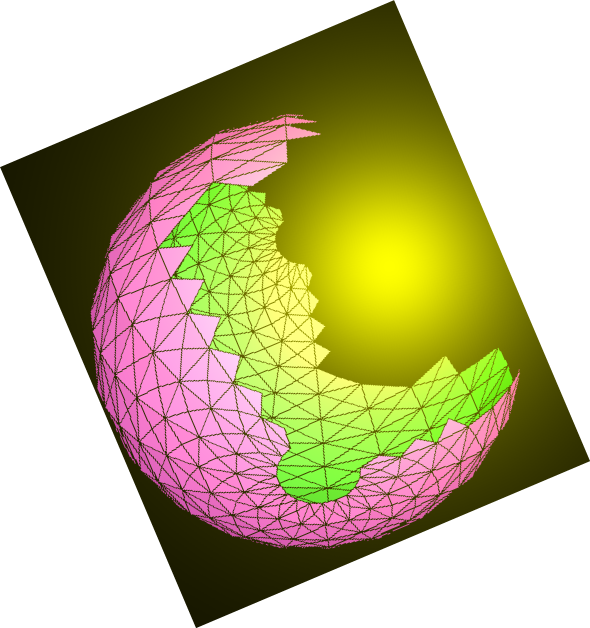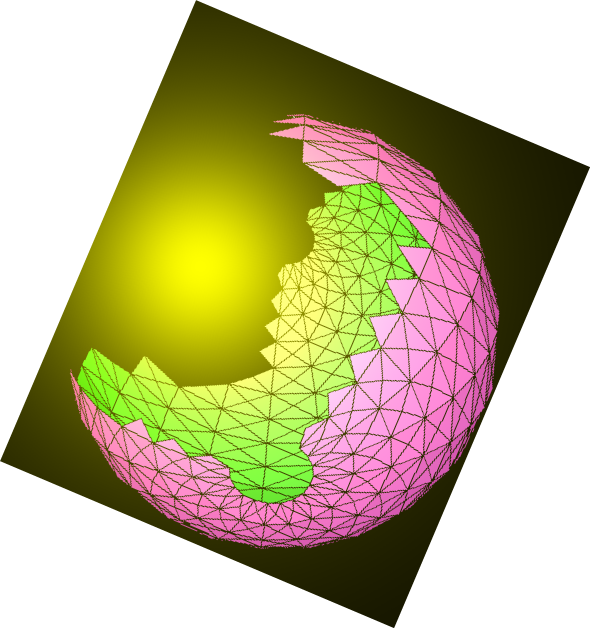# N.S.B. Cosmic Center### High-Energy Algebras by Nazir S. Baaklini

Back to DCSC Books

#### Contents

Introduction: Foundations of high-energy theory. Elementary particle symmetries. Fundamental interactions and gauge principles.

Matrix Formalities: Basic operations. Functions. Conjugation. Invariants. Eigenvalue spectrum and fundamental relation. Infinitesimal variations. Completeness. Decomposition. Traceless and hermitian matrices. External multiplication and composite matrices. Supermatrices.

Tensor Calculus: Operations on tensors. Irreducible tensors. Young tableaux. Multiplicities. Decomposition of tensor products into irreducible parts. Tensor decomposition into subspace components. Totally antisymmetric constant pseudotensors. Coordinate transformations. Space metric. Exterior forms. Invariant integration. Infinitesimal variations.

Spinor Spaces: Clifford akgebras. Dirac algebras. Irreducible decomposition of Dirac-algebraic products. Representations of Dirac and Majorana matrices. Transpositions of Dirac algebras.

Algebraic Transormations: Simple algebras. Angular momentum algebra. Classification of simple algebras. Representation theory of simple algebras. Algebraic transformations and infinitesimal variations.

Orthogonal Algebras: The SO(n) algebra. Tensorial representations. Adjoint multiplet. Spinorial representations. Irreducible spinors. Tensorial decomposition of spinorial products. Pseudo-orthogonal transormations. Orthogonal decomposition of orthogonal algebras.

Unitary Algebras: Special unitary transormations. Matrix relations. The SU(n) algebra. Tensorial representations. Adjoint multiplet. Young tableaux. Isomorphic unitary and orthogonal algebras. Unitary decomposition of SU(n). Unitary decomposition of SO(2n).

Symplectic Algebras: Tensorial representations. Symplectic decomposition. Unitary decomposition of SP(2n). Fundamental multiplet. Invariant bilinear form. Adjoint multiplet. Adjoint bilinear Invariant. Invariant bilinear operator. Symplectic relationships to orthogonal algebras.

Exceptional Algebra G2: G2 and SU(3). The G2 Algebra. Invariant bilinear operator. Fundamental multiplet. Invariant bilinear form. Antisymmetric form. Adjoint multiplet. Adjoint bilinear invariant. Adjoint form multiplet. The 27-dimensional multiplet. Relationship of G2 to SU(2)xSU(2). Relationship of O(7) to G2.

Exceptional Algebra F4: F4 and O(9). Fundamental representation and the F4 algebra. Invariant bilinear form. Symmetric form. Adjoint multiplet. Adjoint invariant. Antisymmetric adjoint form. The 273-dimensional multiplet. The 324-dimensional multiplet. Relationship of F4 to SU(3)xSU(3). Relationship of F4 to SU(4)xSU(2). Relationship of F4 to SP(6)xSU(2). Relationship of F4 to G2xSU(2).

Exceptional Algebra E6: E6 and O(10). Fundamental representation and the E6 algebra. Invariant operator. Invariant bilinear form. Symmetric form. Adjoint multiplet. Adjoint bilinear invariant. Adjoint form. The 351-dimensional multiplet. The other 351-dimensional multiplet. The 650-dimensional multiplet. Relationship of E6 to SU(6)xSU(2). Relationship of E6 to SU(3)xSU(3)xSU(3). Relationship of E6 to G2xSU(3).

Exceptional Algebra E7: E7 and SU(8). Fundamental representation and the E7 algebra. Invariant bilinear operator. Bilinear invariant. Adjoint multiplet. Adjoint invariant. Symmetric adjoint form. Relationship of E7 to SU(6)xSU(3). Relationship of E7 to O(12)xSU(2). Relationship of E7 to E6. Relationship of E7 to F4xSU(2), Relationship of E7 to G2xSP(6).

Exceptional Algebra E8: The E8 algebra and SU(9). Jacobi identities. Adjoint multiplet. Invariant bilinear form. Invariant bilinear operator. The E8 algebra and SO(16). The 3875-dimensional multiplet. Relationship of E8 to SU(5)xSU(5). Relationship of E8 to E7xSU(2). Relationship of E8 to E6xSU(2). Relationship of E8 to F4xG2.

Superalgebras: Supertransformations and superparameters. Jacobi identities. Algebra of variations. Representation and classification of simple superalgebras. Unitary superalgebras. Fundamental SU(n|m) supermultiplet. Adjoint SU(n|m) supermultiplet. Orthosymplectic superalgebras. Exceptional superalgebras.

Spacetime Algebra: Poincare algebra. Representations of the Lorentz subalgebra. Dirac algebra in 4-dimensional spacetime. Lorentz spinors. Representations of the Poincare algebra. Relativistic fields.

Extended Spacetime Algebra: The conformal algebra. Orthogonal spacetime extension. Symplectic spacetime extension. Unitary spacetime extension. Exceptional spacetime extension.

Spacetime Superlgebras: Poincare superalgebra. Algebra of fermionic generators. Supermultiplets and superfields. Extended Poincare superalgebras. Conformal superalgebras. Orthosymplectic spacetime superalgebras. Unitary spacetime superalgebras. Exceptional spacetime superalgebras.

Supplementary Problems

Useful References

Back to DCSC BooksHome
N.S.B. Cosmic Center by Nazir S. Baaklini